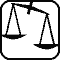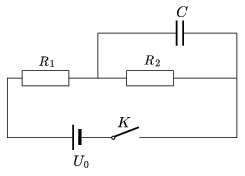Mathematical and Physical Journal
for High Schools
Issued by the MATFUND Foundation
 Already signed up? New to KöMaL?

# Exercises and problems in PhysicsJanuary 2005## Experimental problem

M. 257. Determine the collision number of the central linear collision of two identical coins. Execute the measurement with different coin pairs too.

(6 points)## Theoretical problems

It is allowed to send solutions for any number of problems, but final scores of students of grades 9-12 are computed from the 5 best score in each month. Final scores of students of grades 1-8 are computed from the 3 best scores in each month.

P. 3763. In a car handling contest the task is to go along a straight road without spinning the wheels. The winner is the person who stops exactly at distance s in the shortest time. What can the world record be if the coefficient of the sticking friction between the tyres and the road is $\displaystyle \mu$0?

(4 points)

P. 3764. On which part of a window can frost-work develop if the window is a) an Italian single pane window? b) a Hungarian double pane window? c) a Swedish triple pane window?

(4 points)

P. 3765. In two glasses there is liquid material of the same type, the volume of one is V1, its temperature of is T1, the volume of the other is V2 and its temperature is T2. We mix the liquids and wait while the temperature settles. What is the common temperature if we neglect any heat loss?

(4 points)

P. 3766. A certain amount of ideal gas goes through the thermal cycle shown in the figure. States A and B are fixed, C can change, but the CA process must be isobaric. a) What is the maximum effective work done in the cycle if the temperature is decreasing all along process BC? b) What is the efficiency of the machine doing this thermal cycle in this case?(5 points)

P. 3767. At the session of the Royal Society on 4 December 1679, Hooke, the Secretary, read the following letter sent by Newton:

Scholastic scientists, attempting to prove the absurdity of the statement according to which the Earth would rotate around its axis, argued as follows. Let us drop a heavy body from the top of a tower. If the Earth indeed rotated from the west to the east, the Earth under it would turn to the east during the falling, and the body would be found not at the bottom of the tower, but westwards from it. This argument is quite wrong, writes Newton, since it does not take into account the initial velocity of the body. As the top of the tower is at a larger distance than its bottom, the initial velocity of the body is actually larger then down below at the bottom. The body would be found, therefore, not to the west, but to the east from the vertical line, dropped from the top of the tower.

Where will the body be found? (Let us assume for simplicity that the tower is on the Equator.)

(5 points)

P. 3768. In the connection shown in the figure switch K is initially open and the capacitor is uncharged. Then we close the switch and let the big capacitor to be charged fully up and then we open the switch again. Determine the currents in the resistances a) immediately after closing the switch. b) a long time after closing the switch. c) immediately after opening the switch. (Data: U0=30 V; R1=10 k$\displaystyle \Omega$; R2=5 k$\displaystyle \Omega$.)(5 points)

P. 3769. There is a 0.5 $\displaystyle \mu$A proton beam coming from a 30 MeV cyclotron. a) How many protons impact into the target in one second? b) How much energy is carried to the target in 10 seconds by the proton beam if the protons remain in the target? c) How much would the temperature of the 0.01 kg leaden target rise in 10 seconds if heat removal were not ensured.

(4 points)

P. 3770. A large concave mirror with a radius of curvature of 0.5 m is placed on a horizontal plate. We pour a small amount of water into the mirror and place on it a plane-convex lens with a radius of curvature of 1.0 m whose plane side just covers the water. Then we place a point-like light source above this set-up at a height of 0.3 m. Where does the image of the light source develop? (The refractive index of water is 1.33 and the refractive index of glass is 1.50.)

(5 points)

P. 3771. Observed from Pluto its satellite Charon can always be seen in the same place (Plutostationary'' satellite). Between two subsequent sunrises on Pluto 6 terrestrial days and 9 hours pass. The radius of Charon's orbit is about 8.5 times the diameter of Pluto. Give an estimation of the density of Pluto.

(4 points)